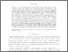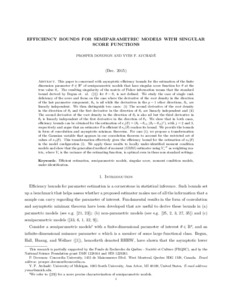Title:

# EFFICIENCY BOUNDS FOR SEMIPARAMETRIC MODELS WITH SINGULAR SCORE FUNCTIONS

Dovonon, Prosper and Atchade, F. Yves (2015) EFFICIENCY BOUNDS FOR SEMIPARAMETRIC MODELS WITH SINGULAR SCORE FUNCTIONS. (Submitted)Preview
Text (application/pdf)
EfficiencyGMM.pdf
263kB

## Abstract

This paper is concerned with asymptotic efficiency bounds for the estimation of the finite dimension parameter $\theta\in \rset^p$ of semiparametric models that have singular score function for $\theta$ at the true value $\theta_\star$. The resulting singularity of the matrix of Fisher information means that the standard bound derived by Begun et. al. () for $\theta-\theta_\star$ is not defined. We study the case of single rank deficiency of the score and focus on the case where the derivative of the root density in the direction of the last parameter component, $\theta_2$, is nil while the derivatives in the $p-1$ other directions, $\theta_1$, are linearly independent. We then distinguish two cases: (i) The second derivative of the root density in the direction of $\theta_2$ and the first derivative in the direction of $\theta_1$ are linearly independent and (ii) The second derivative of the root density in the direction of $\theta_2$ is also nil but the third derivative in $\theta_2$ is linearly independent of the first derivative in the direction of $\theta_1$.

We show that in both cases, efficiency bounds can be obtained for the estimation of $\kappa_j(\theta)=(\theta_1-\theta_{\star1}, (\theta_2-\theta_{\star2})^j)$, with $j=2$ and 3, respectively and argue that an estimator $\hat{\theta}$ is efficient if $\kappa_j(\hat{\theta})$ reaches its bound. We provide the bounds in form of convolution and asymptotic minimax theorems. For case (i), we propose a transformation of the Gaussian variable that appears in our convolution theorem to account for the restricted set of values of $\kappa_2(\theta)$. This transformation effectively gives the efficiency bound for the estimation of $\kappa_2(\theta)$ in the model configuration (i). We apply these results to locally under-identified moment condition models and show that the generalized method of moment (GMM) estimator using $V_\star^{-1}$ as weighting matrix, where $V_\star$ is the variance of the estimating function, is optimal even in these non standard settings.

Divisions: Concordia University > Faculty of Arts and Science > Economics Article No Dovonon, Prosper and Atchade, F. Yves December 2015 980803 PROSPER DOVONON 28 Jan 2016 16:36 18 Jan 2018 17:52
All items in Spectrum are protected by copyright, with all rights reserved. The use of items is governed by Spectrum's terms of access.

Repository Staff Only: item control pageResearch related to the current document (at the CORE website)
Back to top